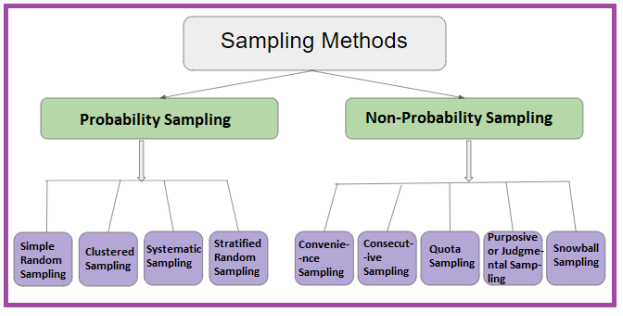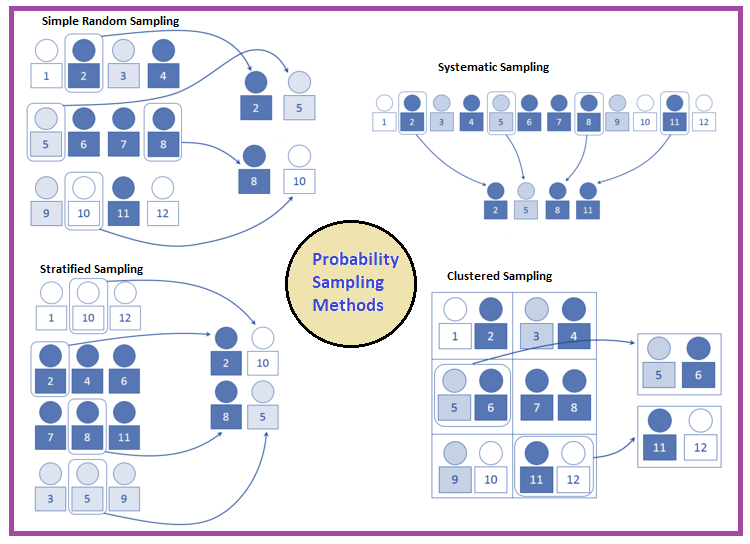# Sampling Methods

In Statistics, the sampling method is the process of studying the population by gathering information and analyzing those data. It is the basis of the data where the sample space is enormous. The statistical research is of two forms:

• In the first form, each domain is studied, and the result can be obtained by computing the sum of all units.
• In the second form, only a unit in the field of the survey is taken. It represents the domain. The result of these samples extends to the domain. This type of study is known as the sample survey.

In this article, let us discuss the different sampling methods in research such as probability sampling and non-probability sampling methods and various methods involved in those two approaches in detail.

## Types of Sampling Method

In Statistics, there are different sampling techniques available to get relevant results from the population. The two different types of sampling methods are::

• Probability Sampling
• Non-probability Sampling## What is Probability Sampling?

The probability sampling method utilizes some form of random selection. In this method, all the eligible individuals have a chance of selecting the sample from the whole sample space. This method is more time consuming and expensive than the non-probability sampling method. The benefit of using probability sampling is that it guarantees the sample that should be the representative of the population.

### Probability Sampling Types

Probability Sampling methods are further classified into different types, such as simple random sampling, systematic sampling, stratified sampling, and clustered sampling. Let us discuss the different types of probability sampling methods here in detail.

### Simple Random Sampling

In simple random sampling technique, every item in the population has an equal and likely chance of being selected in the sample. Since the item selection entirely depends on the chance, this method is known as “Method of chance Selection”. As the sample size is large, and the item is chosen randomly, it is known as “Representative Sampling”.

### Systematic Sampling

In the systematic sampling method, the items are selected from the target population by selecting the random selecting point and selecting the other methods after a fixed sample interval. It is calculated by dividing the total population size by the desired population size.

### Stratified Sampling

In a stratified sampling method, the total population is divided into smaller groups to complete the sampling process. The small group is formed based on a few characteristics in the population. After separating the population into a smaller group, the statisticians randomly select the sample.

### Clustered Sampling

In the clustered sampling method, the cluster or group of people are formed from the population set. The group has similar significatory characteristics. Also, they have an equal chance of being a part of the sample. This method uses simple random sampling for the cluster of population.

All these four methods can be understood in a better manner with the help of the figure given below. The figure contains various examples of how samples will be taken from the population using different techniques.## What is Non-Probability Sampling?

The non-probability sampling method is a technique in which the researcher selects the sample based on subjective judgment rather than the random selection. In this method, not all the members of the population have a chance to participate in the study.

### Non-Probability Sampling Types

Non-probability Sampling methods are further classified into different types, such as convenience sampling, consecutive sampling, quota sampling, judgemental sampling, snowball sampling. Here, let us discuss all these types of non-probability sampling in detail.

### Convenience Sampling

In a convenience sampling method, the samples are selected from the population directly because they are conveniently available for the researcher. The samples are easy to select, and the researcher did not choose the sample that outlines the entire population.

### Consecutive Sampling

Consecutive sampling is similar to convenience sampling with a slight variation. The researcher picks a single person or a group of people for sampling. Then the researcher researches for a period of time to analyze the result and move to another group if needed.

### Quota Sampling

In the quota sampling method, the researcher forms a sample that involves the individuals to represent the population that is based on specific traits or qualities. The researcher chooses the sample subsets that bring the useful collection of data that generalizes the entire people.

### Purposive or Judgmental Sampling

In purposive sampling, the samples are selected only based on the researcher’s knowledge. As their knowledge is instrumental in creating the samples, there are the chances of obtaining highly accurate answers with a minimum marginal error. It is also known as judgmental sampling or authoritative sampling.

### Snowball Sampling

The snowball sampling is also known as chain-referral sampling technique. In this method, the samples have traits that are difficult to find. So, each identified member of a population is asked to find the other sampling units. Those sampling units also belong to the same targeted population.

For more information on Statistics concepts, stay tuned with BYJU’S- The Learning App and explore more videos.